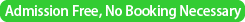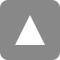﻿ 第12回統計的機械学習セミナー / 12th Statistical Machine Learning Seminar | 統計数理研究所

# 第12回統計的機械学習セミナー / 12th Statistical Machine Learning Seminar

Date&Time
July 11th (Thu) 2013　15:00-17:00Place
Seminar Room 5 (3F, D313), Institute of Statistical Mathematics (Tachikawa, Tokyo)Speaker 1
Vincent Q. Vu
(Dept. Statistics, Ohio State University)
http://www.vince.vu/
Title
Sparse Principal Components and Subspaces : Concepts, Theory, and Algorithms
Abstract

Principal components analysis (PCA) is a popular technique for unsupervised dimension reduction that has a wide range of application in science, engineering, and any place where multivariate data is abundant. Its main idea is to look for linear combinations of the variables with the largest variance. These linear combinations correspond to eigenvectors of a covariance matrix. However, in modern applications where the number of variables can be much larger than the number of samples, PCA suffers from two major
weaknesses: 1) the interpretability and subsequent use of the principal directions is hindered by their dependence on all of the variables; 2) it is generally inconsistent in high-dimensions, i.e.
the estimated principal directions can be noisy and unreliable.
This has motivated much research over the past decade into a class of techniques called sparse PCA that combine the essence of PCA with the assumption that the phenomena of interest depend mostly on a few variables.

In this talk, I will present some recent theoretical results on sparse PCA including optimal minimax bounds for estimating the principal eigenvector and optimal minimax bounds for estimating the principal subspace spanned by the eigenvectors of a general covariance matrix. The optimal estimators turn out to be NP-hard to compute. However, I will also present a very recent result that shows that a convex relaxation, due to d'Aspremont et al. (2007), is a near-optimal estimator of the principal eigenvector under very general conditions.Speaker 2
Mauricio Alvarez
(Dept. Electrical Engineering, Universidad Tecnológica de Pereira, Columbia)